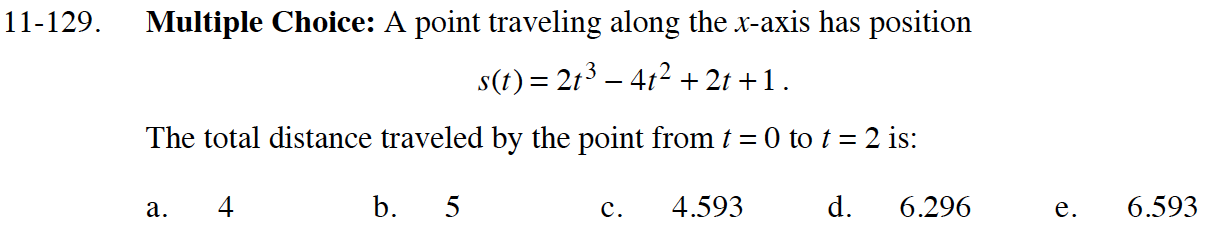### Home > CALC > Chapter 11 > Lesson 11.4.1 > Problem11-129

11-129.Take the derivative and use it to determine when the point changes directions.

The point changes direction when t = 1/3 and t = 1.

$\text{total distance }=s\Big(\frac{1}{3}\Big)-s(0)-\Bigg(s(1)-s\Big(\frac{1}{3}\Big)\Bigg)+s(2)-s(1)$